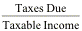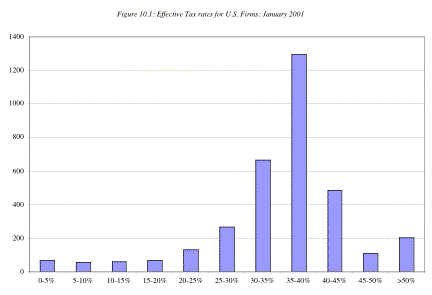More on effective tax rates

To compute the after-tax operating income, you multiply the earnings before interest and taxes by an estimated tax rate. This simple procedure can be complicated by three issues that often arise in valuation. The first is the wide differences you observe between effective and marginal tax rates for these firms and the choice you face between the two in valuation. The second issue arises usually with younger firms and is caused by the large losses they often report, leading to large net operating losses that are carried forward and can save taxes in future years. The third issue arises from the capitalizing of research and development and other expenses. The fact that these expenditures can be expensed immediately leads to much higher tax benefits for the firm.

Effective versus Marginal Tax rate

You are faced with a choice of several different tax rates. The most widely reported tax rate in financial statements is the effective tax rate, which is computed from the reported income statement.

Effective Tax Rate =The second choice on tax rates is the marginal tax rate, which is the tax rate the firm faces on its last dollar of income. This rate depends on the tax code and reflects what firms have to pay as taxes on their marginal income. In the United States, for instance, the federal corporate tax rate on marginal income is 35%; with the addition of state and local taxes, most firms face a marginal corporate tax rate of 40% or higher.

While the marginal tax rates for most firms in the United States should be fairly similar, there are wide differences in effective tax rates across firms. Figure 10.1 provides a distribution of effective tax rates for firms in the United States in January 2001.Note that the number of firms reporting effective tax rates of less than 10% as well as the number of firms reporting effective tax rates of more than 100%. In addition, it is worth noting that this table does not include about 2000 firms that did not pay taxes during the most recent financial year or have a negative effective tax rate.

Reasons for Differences between Marginal and Effective Tax Rates

Given that most of the taxable income of publicly traded firms is at the highest marginal tax bracket, why would a firm’s effective tax rate be different from its marginal tax rate? There are at least three reasons:

1.     Many firms, at least in the United States, follow different accounting standards for tax and reporting purposes. For instance, firms often use straight line depreciation for reporting purposes and accelerated depreciation for tax purposes. As a consequence, the reported income is significantly higher than the taxable income, on which taxes are based.

2.     Firms sometimes use tax credits to reduce the taxes they pay. These credits, in turn, can reduce the effective tax rate below the marginal tax rate.

3.     Finally, firms can sometimes defer taxes on income to future periods. If firms defer taxes, the taxes paid in the current period will be at a rate lower than the marginal tax rate. In a later period, however, when the firm pays the deferred taxes, the effective tax rate will be higher than the marginal tax rate.

4.     The structure of the tax rates is tiered with the first \$X in income taxed at a lower rate (15%), the subsequent \$Y in income taxed at a higher rate (?%) and any amount over \$Z taxed at 35%.  As a result, the effective tax rate based on the total tax a firm pays will be lower than the marginal tax rate which is 35%.

Marginal Tax Rates for Multinationals

When a firm has global operations, its income is taxed at different rates in different locales. When this occurs, what is the marginal tax rate for the firm? There are three ways in which we can deal with different tax rates.

• The first is to use a weighted average of the marginal tax rates, with the weights based upon the income derived by the firm from each of these countries. The problem with this approach is that the weights will change over time if income is growing at different rates in different countries.
• The second is to use the marginal tax rate of the country in which the company is incorporated, with the implicit assumption being that the income generated in other countries will eventually have to be repatriated to the country of origin, at which point the firm will have to pay the marginal tax rate. This assumes that the home country has the highest marginal tax rate of all other countries.
• The third and safest approach is to keep the income from each country separate and apply a different marginal tax rate to each income stream.

Effects of Tax Rate on Value

In valuing a firm, should you use the marginal or the effective tax rates? If the same tax rate has to be applied to earnings every period, the safer choice is the marginal tax rate because none of the reasons noted above can be sustained in perpetuity. As new capital expenditures taper off, the difference between reported and tax income will narrow; tax credits are seldom perpetual; and firms eventually do have to pay their deferred taxes. There is no reason, however, why the tax rates used to compute the after-tax cash flows cannot change over time. Thus, in valuing a firm with an effective tax rate of 24% in the current period and a marginal tax rate of 35%, you can estimate the first year’s cash flows using the effective tax rate of 24% and then increase the tax rate to 35% over time. It is critical that the tax rate used in perpetuity to compute the terminal value be the marginal tax rate.

When valuing equity, we often start with net income or earnings per share, which are after-tax earnings. While it looks like we can avoid dealing with the estimating of tax rates when using after-tax earnings, appearances are deceptive. The current after-tax earnings of a firm reflect the taxes paid this year. To the extent that tax planning or deferral caused this payment to be very low (low effective tax rates) or very high (high effective tax rates), we run the risk of assuming that the firm can continue to do this in the future if we do not adjust the net income for changes in the tax rates in future years.

The Effect of Net Operating Losses

For firms with large net operating losses carried forward or continuing operating losses, there is the potential for significant tax savings in the first few years that they generate positive earnings. There are two ways of capturing this effect.

One is to change tax rates over time. In the early years, these firms will have a zero tax rate as losses carried forward offset income. As the net operating losses decrease, the tax rates will climb toward the marginal tax rate. As the tax rates used to estimate the after-tax operating income change, the rates used to compute the after-tax cost of debt in the cost of capital computation also need to change. Thus, for a firm with net operating losses carried forward, the tax rate used for both the computation of after-tax operating income and cost of capital will be zero during the years when the losses shelter income.

The other approach is often used when valuing firms that already have positive earnings but have a large net operating loss carried forward. Analysts will often value the firm, ignoring the tax savings generated by net operating losses, and then add to this amount the expected tax savings from net operating losses. Often, the expected tax savings are estimated by multiplying the tax rate by the net operating loss. The limitation of doing this is that it assumes that the tax savings are both guaranteed and instantaneous. To the extent that firms have to generate earnings to create these tax savings and there is uncertainty about earnings, it will over estimate the value of the tax savings.

There are two final points that needs to be made about operating losses. To the extent that a potential acquirer can claim the tax savings from net operating losses sooner than the firm generating these losses, there can be potential for tax synergy that we will examine in the chapter on acquisitions. The other is that there are countries where there are significant limitations in how far forward or back operating losses can be applied. If this is the case, the value of these net operating losses may be curtailed.

 A negative effective tax rate usually arises because a firm is reporting an income in its tax books (on which it pays taxes) and a loss in its reporting books.

 Since the effective tax rate is based upon the taxes paid (which comes from the tax statement), the effective tax rate will be lower than the marginal tax rate for firms that change accounting methods to inflate reported earnings.